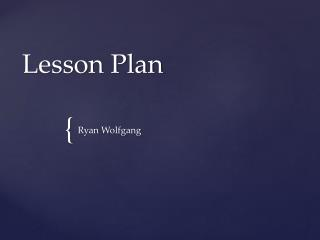DownloadDownload PresentationLesson Plan

# Lesson Plan

Télécharger la présentation## Lesson Plan

- - - - - - - - - - - - - - - - - - - - - - - - - - - E N D - - - - - - - - - - - - - - - - - - - - - - - - - - -
##### Presentation Transcript

1. Lesson Plan Ryan Wolfgang

2. 1. Use Polar Coordinates to specify locations on a plane • 2. Represent translations using vectors • 3. Describe Multiplication of a vector and a scalar graphically and algebraically, and apply to problem situations • 4. Use trigonometric relationships to determine lengths and angle measures; i.e., Law of Sines and Law of Cosines • 5. Identify, sketch and classify the cross sections of three dimensional objects Academic Content Standards

3. The students will be able to solve triangles using the Law of Sines and Law of Cosines • Be able to solve word problems in which they have to draw their own triangle • ex: An Aircraft Carrier Leaves Pearl Harbor Port in Hawaii and heads west at 28 mph. A helicopter is 175 miles from the carrier at an angle of 35 degrees Southwest. a) What angle should the helicopter travel at its cruising speed of 130 mph to meet carrier? b) How long will it take? Objectives

4. First 5 minutes – Collect and discuss previous nights homework if there are any questions • 15-20 minutes – Introduce and lecture about the Law of Sines going over example problems and answering questions • 15-20 minutes - Introduce Law of Cosines and provide example problems • 10 minutes – Group work involving both Laws • 5 minutes - Assign homework and allow to start in class. Lesson Procedure

5. Break up into groups of two or three • I will give each group a problem that involves using both Laws in same problem • They will write out problem on board and I will go through each of them Activity

6. Scientific Calculator(TI-84 or TI-84 Plus recommended) • Pencil….. NO PENS!!!! Materials Needed

7. This lesson can be related to Physics because the same laws are used in finding velocities and directions in Physics • Also Reading can be related because the student has to read and analyze the problems and figure out exactly what the problem is asking. Interdisciplinary Connections

8. I will assign certain problems that I find the most beneficial out of the book and assign 5-7 problems. Due at the beginning of class next day Homework

9. Definintion of Law of Sines - SinA= SinB= SinC a b c • Definition of Law of Cosines c^2 = a^2 + b^2 – 2ab cosC b^2 = a^2 + c^2 – 2ac cosB a^2 = b^2 + c^2 – 2bc cosA Key Vocabulary

10. Scientific Calculators have tons of functions on them that make life easier • Smart board if the school has them • Projector Technology Connections

11. Defranza, James. Precalculus. Mason: Cengage Learning, 2010. Print. • "Experimental Feature." Wolfram|Alpha: Computational Knowledge Engine. Web. 15 May 2012. <http:// www.wolframalpha.com/>. Research Connections